Published

# Purdue Verti-Fix

Our device is for primary care physicians as well as at home patients to treat Benign Paroxysmal Positional Vertigo with ease.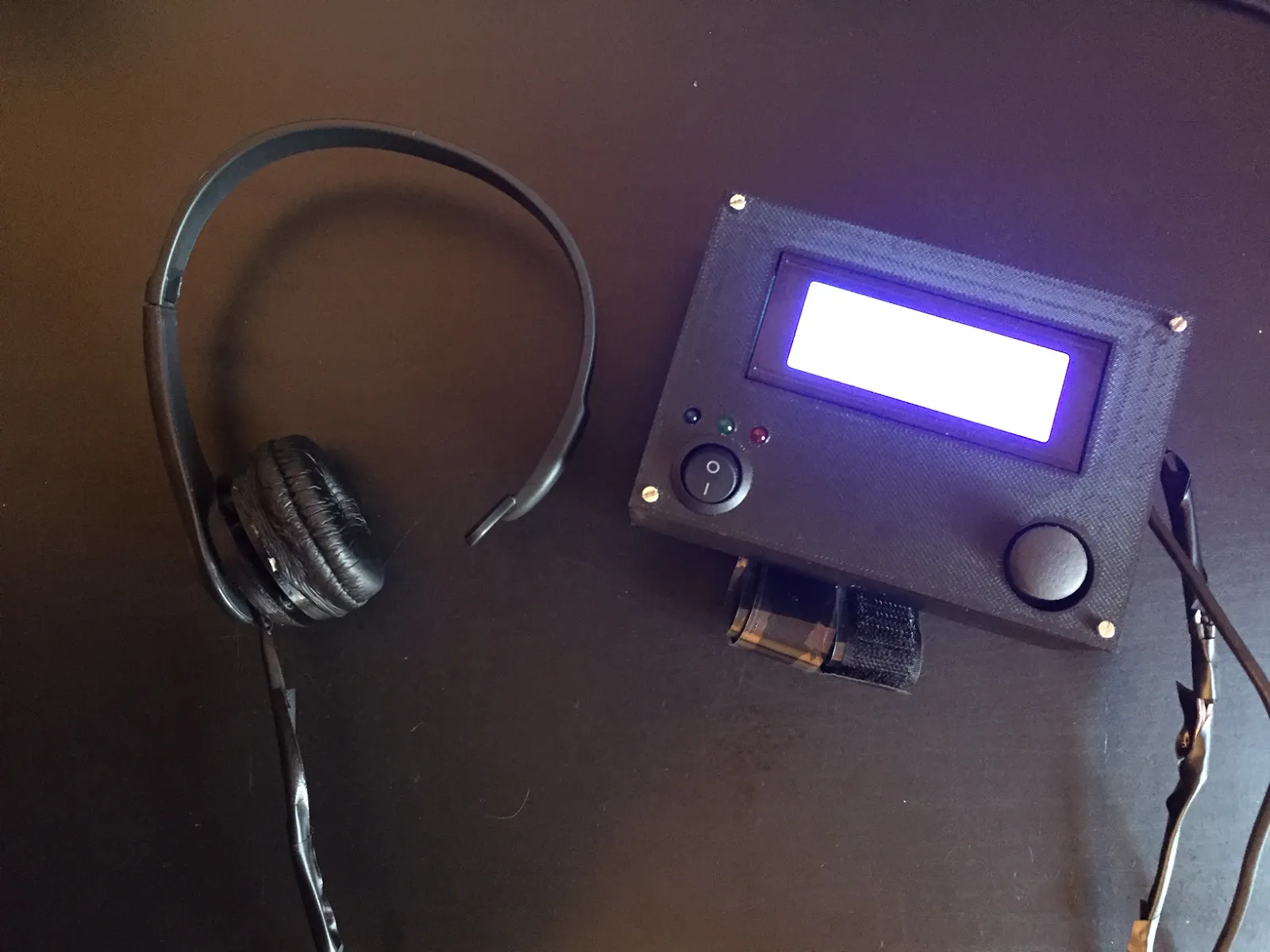## Things used in this project

### Hardware components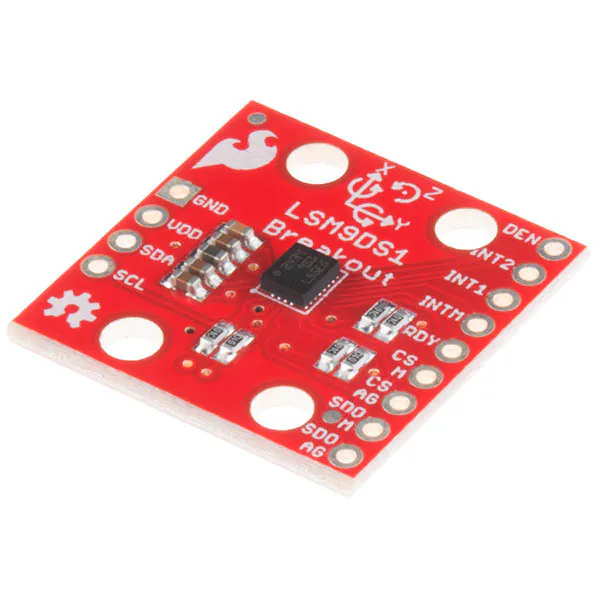SparkFun 9 Degrees of Freedom IMU Breakout - LSM9DS1
×1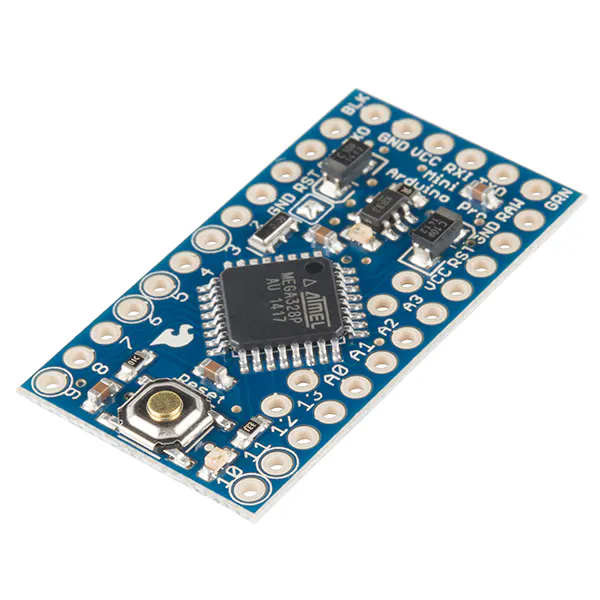SparkFun Arduino Pro Mini 328 - 3.3V/8MHz
×1LED (generic)
×3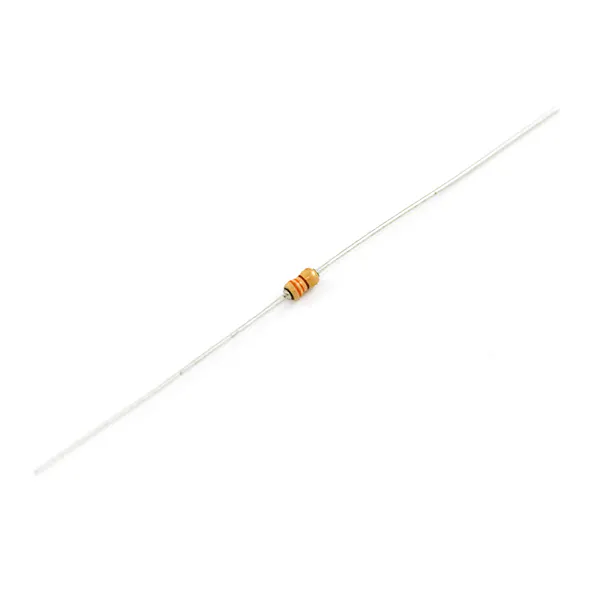Resistor 330 ohm
×3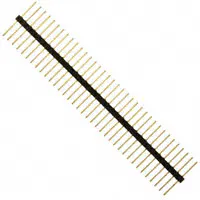Male-Header 36 Position 1 Row- Long (0.1")
×1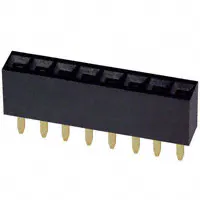Female Header 8 Position 1 Row (0.1")
×6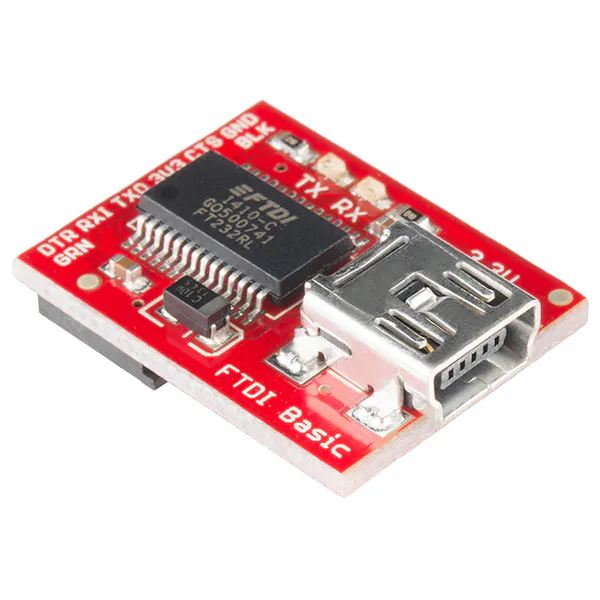SparkFun FTDI Basic Breakout - 3.3V
×1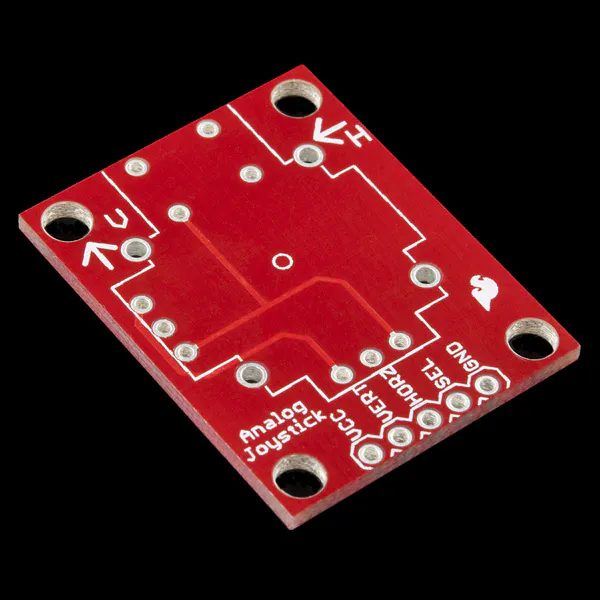SparkFun Thumb Joystick Breakout
×1
 SparkFun Thumb Joystick
×1
 Sony PSEye Camera
×1
 Sony PSEye Camera
×1
 M12 Lens
×1
 M12 Lens Mount
×1
 IR LEDs
×36
 MOSFET N-CH 60V 60A TO-220
×2
 MOSFET N-CH 800V 2.5A
×2
 MOSFET N-CH 75V 120A TO-220
×2Resistor 10k ohm
×1
 Voltage Regulator
×1
 Rocker Switch
×1
 20x4 LCD
×1

### Software apps and online servicesArduino IDE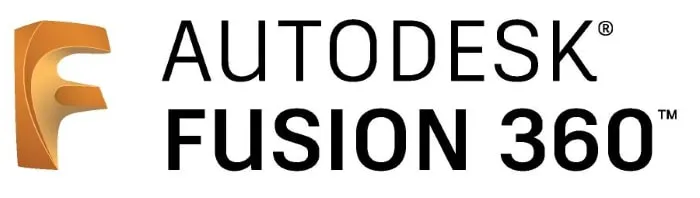Autodesk Fusion 360### Hand tools and fabrication machinesSoldering iron (generic)

## Custom parts and enclosures

### Top Lid

Central Console Top Enclosure

## Schematics

### Eyewriter Schematic

From the Instructables Eyewriter 2.0, we obtained and used this schematic along with the one from Hikari Blogspot to build our eye tracker.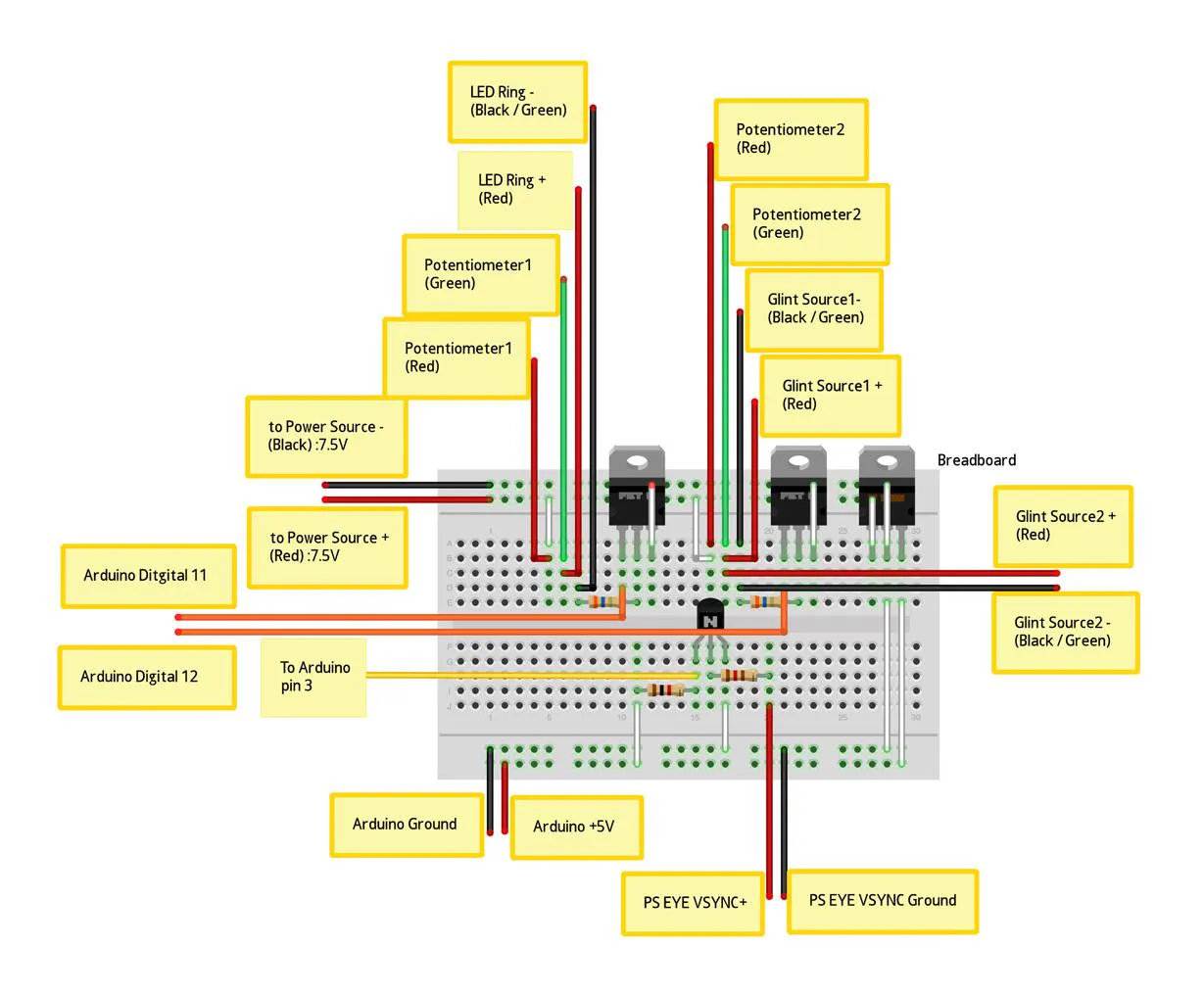### Maneuvers Schematic

This is the schematic for the device that ensures movements are carried out properly.

### Eyetracking Schematic

Includes the PSEye, the ring of infrared LEDs, and the Arduino. This was taken from http://hikarielectronics.blogspot.com/ which details how to build the Eyewriter 2.0 with the PSEye camera.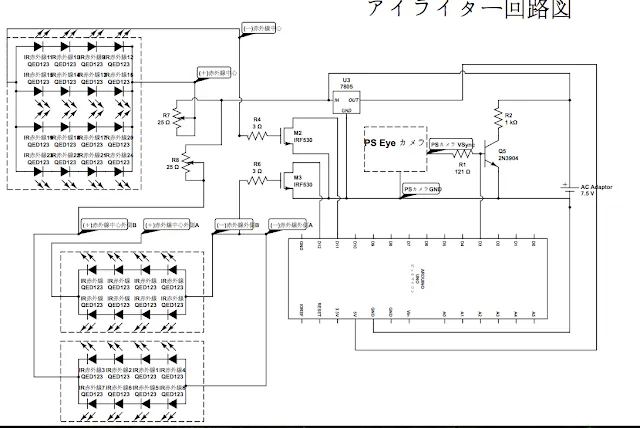## Code

### Arduino Programming

Arduino
This code runs on the microcontroller to carry out the maneuvers required to treat BPPV Vertigo.
```#include <Wire.h>
#include <SPI.h>
#include <SparkFunLSM9DS1.h>
#include <Integrator.h>
#include <Wire.h>
#include <LiquidCrystal_I2C.h>

LSM9DS1 imu;
LiquidCrystal_I2C lcd(0x27, 20, 4);

#define LSM9DS1_M	0x1E // Would be 0x1C if SDO_M is LOW
#define LSM9DS1_AG	0x6B // Would be 0x6A if SDO_AG is LOW

// x - 0
// y - 1
// z - 2
#define FFA 1
#define RFA 2
#define UFA 0

#define GET_FFA(IMU) (FFA == 0 ? IMU.ax : (FFA == 1 ? IMU.ay : (FFA == 2 ? IMU.az : 0)))
#define GET_RFA(IMU) (RFA == 0 ? IMU.ax : (RFA == 1 ? IMU.ay : (RFA == 2 ? IMU.az : 0)))
#define GET_UFA(IMU) (UFA == 0 ? IMU.ax : (UFA == 1 ? IMU.ay : (UFA == 2 ? IMU.az : 0)))

#define GET_FFG(IMU) (FFA == 0 ? IMU.gx : (FFA == 1 ? IMU.gy : (FFA == 2 ? IMU.gz : 0)))
#define GET_RFG(IMU) (RFA == 0 ? IMU.gx : (RFA == 1 ? IMU.gy : (RFA == 2 ? IMU.gz : 0)))
#define GET_UFG(IMU) (UFA == 0 ? IMU.gx : (UFA == 1 ? IMU.gy : (UFA == 2 ? IMU.gz : 0)))

#define GET_FFM(IMU) (FFA == 0 ? IMU.mx : (FFA == 1 ? IMU.my : (FFA == 2 ? IMU.mz : 0)))
#define GET_RFM(IMU) (RFA == 0 ? IMU.mx : (RFA == 1 ? IMU.my : (RFA == 2 ? IMU.mz : 0)))
#define GET_UFM(IMU) (UFA == 0 ? IMU.mx : (UFA == 1 ? IMU.my : (UFA == 2 ? IMU.mz : 0)))

#define POSITION_MET 9
#define LED2 8
#define LED3 7

#define PROGRAM_BUTTON 6
#define SAVE_STATE_BUTTON 5

struct Measurement{
double front_facing;
double right_facing;
double up_facing;
};

enum PositionMatch{
POSITION_MATCH,
POSITION_NOT_MATCH,
POSITION_UNSURE,
};

enum PositionType{
POSITION_SITTING,
POSITION_LAYING,
};

Measurement gyro_reference{0, 0, 0};

#define MEASUREMENT_CYCLES 1
Measurement takeMeasurementAccel(int measurement_cycles)
{
Measurement average {0, 0, 0};

for(int i = 0; i < measurement_cycles; i++){
if ( imu.accelAvailable() )
{
}
/*
vector g_raw(imu.calcAccel(imu.ax), imu.calcAccel(imu.ay), imu.calcAccel(imu.az));
*/
average.front_facing += imu.calcAccel(GET_FFA(imu));
average.right_facing += imu.calcAccel(GET_RFA(imu));
average.up_facing += imu.calcAccel(GET_UFA(imu));
}

average.front_facing /= measurement_cycles;
average.right_facing /= measurement_cycles;
average.up_facing /= measurement_cycles;

return average;
}

Measurement takeMeasurementAccel(){
return takeMeasurementAccel(MEASUREMENT_CYCLES);
}

Measurement takeMeasurementGyro(int measurement_cycles)
{
Measurement average {0, 0, 0};

for(int i = 0; i < measurement_cycles; i++){
if ( imu.gyroAvailable() )
{
}
/*
vector g_raw(imu.calcAccel(imu.ax), imu.calcAccel(imu.ay), imu.calcAccel(imu.az));
*/
average.front_facing += imu.calcGyro(GET_FFG(imu));
average.right_facing += imu.calcGyro(GET_RFG(imu));
average.up_facing += imu.calcGyro(GET_UFG(imu));
}

average.front_facing /= measurement_cycles;
average.right_facing /= measurement_cycles;
average.up_facing /= measurement_cycles;

average.front_facing -= gyro_reference.front_facing;
average.right_facing -= gyro_reference.right_facing;
average.up_facing -= gyro_reference.up_facing;

return average;
}

Measurement takeMeasurementGyro(){
return takeMeasurementGyro(MEASUREMENT_CYCLES);
}

Measurement takeMeasurementMag(int measurement_cycles)
{
Measurement average {0, 0, 0};

for(int i = 0; i < measurement_cycles; i++){
if ( imu.magAvailable() )
{
}
/*
vector g_raw(imu.calcAccel(imu.ax), imu.calcAccel(imu.ay), imu.calcAccel(imu.az));
*/
average.front_facing += imu.calcMag(GET_FFM(imu));
average.right_facing += imu.calcMag(GET_RFM(imu));
average.up_facing += imu.calcMag(GET_UFM(imu));
}

average.front_facing /= measurement_cycles;
average.right_facing /= measurement_cycles;
average.up_facing /= measurement_cycles;

return average;
}

Measurement takeMeasurementMag(){
return takeMeasurementMag(MEASUREMENT_CYCLES);
}

void calibrateGyro(){
gyro_reference = takeMeasurementGyro(100);
}

#define JOYSTICK_BUTTON 6
#define JOYSTICK_HORIZONTAL A0
#define JOYSTICK_VERTICAL A1

//MUST BE ZERO INITIALIZED!!!

void setup()
{
lcd.init();
lcd.backlight();

pinMode(JOYSTICK_BUTTON, INPUT);
pinMode(JOYSTICK_HORIZONTAL, INPUT);
pinMode(JOYSTICK_VERTICAL, INPUT);

Serial.begin(115200);
Serial.setTimeout(0);

// Before initializing the IMU, there are a few settings
// we may need to adjust. Use the settings struct to set
// the device's communication mode and addresses:
imu.settings.device.commInterface = IMU_MODE_I2C;
// The above lines will only take effect AFTER calling
// imu.begin(), which verifies communication with the IMU
// and turns it on.
if (!imu.begin())
{
Serial.println("Failed to communicate with LSM9DS1.");
Serial.println("Double-check wiring.");
Serial.println("Default settings in this sketch will " \
"work for an out of the box LSM9DS1 " \
"Breakout, but may need to be modified " \
"if the board jumpers are.");
while (1)
;
}
//Wire.setClock(400000);
/*
reference_chin = getDegreesChinTilt();
reference_neck = getDegreesNeck();
reference_spin = getMaxSpin();
*/
/*
getChinTilt = &getDegreesChinTilt;
getNeckTilt = &getDegreesNeck;
*/
/*
getGyroData = &takeMeasurementGyro;
getAccelData = &takeMeasurementAccel;
*/
calibrateGyro();
}
/*
double getDegreesNeck(const Measurement& m){
return atan2(m.right_facing, pow(pow(m.front_facing, 2) + pow(m.up_facing, 2), .5)) * 180.0 / M_PI;
}

double calcDegreesChinTilt(const Measurement& m){
return atan2(m.up_facing, pow(pow(m.front_facing, 2) + pow(m.right_facing, 2), .5)) * 180.0 / M_PI;
}
double getDegreesNeck(){
return getDegreesNeck(takeMeasurementAccel()) - reference_neck;
}

double getDegreesChinTilt(){
return calcDegreesChinTilt(takeMeasurementAccel()) - reference_chin;
}
*/
double calcMaxSpin(const Measurement& m){
if(m.front_facing > m.right_facing && m.front_facing > m.up_facing)
return m.front_facing;
else if(m.right_facing > m.front_facing && m.right_facing > m.up_facing)
return m.right_facing;
else if(m.up_facing > m.front_facing && m.up_facing > m.right_facing)
return m.up_facing;
else
return m.up_facing;
}

double getMaxSpin(){
return calcMaxSpin(takeMeasurementGyro()) - calcMaxSpin(gyro_reference);
}

double compass(){
Measurement m = takeMeasurementMag();
/*
Serial.print(m.right_facing);
Serial.print(", ");
Serial.print(m.front_facing);
Serial.println();
*/
return atan2(m.right_facing, m.front_facing)*180/PI;
}

char digitToChar(int digit){
switch(digit){
case 0:
return '0';
case 1:
return '1';
case 2:
return '2';
case 3:
return '3';
case 4:
return '4';
case 5:
return '5';
case 6:
return '6';
case 7:
return '7';
case 8:
return '8';
case 9:
return '9';
}
return '\0';
}

void numToChars(char* buff, int number, int max_characters){
int buffer_pointer = max_characters - 1;

Serial.print("---> ");
Serial.println(number);
while(buffer_pointer >= 0 && number > 0){
buff[buffer_pointer] = digitToChar(number % 10);
Serial.print(buffer_pointer);
Serial.print(" ");
Serial.println(digitToChar(number % 10));
number /= 10;

buffer_pointer--;
}
}

void writeStep(int num, int total){
lcd.clear();
lcd.setCursor(0, 0);

char buff = {' ', ' ', ' ', ' '};
buff = '\0';

lcd.print("Step ");
numToChars(buff, num, 2);
lcd.print(buff);
lcd.print("/");
numToChars(buff, total, 2);
lcd.print(buff);
}

void writeInstruction(const char* instruction1, const char* instruction2){
lcd.setCursor(0, 1);
lcd.print(instruction1);

lcd.setCursor(0, 2);
lcd.print(instruction2);
}

void writeUtility(const char* utility, int value){
char buff = {' ', ' ', ' ', ' '};
buff = '\0';

lcd.setCursor(0, 3);
lcd.print(utility);
lcd.print(" ");
numToChars(buff, value, 2);
lcd.print(buff);

}

void writeError(const char* error1, const char* error2){
lcd.clear();

lcd.setCursor(0, 0);
lcd.print("Error!");
lcd.setCursor(0, 1);
lcd.print(error1);
lcd.setCursor(0, 2);
lcd.print(error2);
}

#define NO_ACCEL_THRESHOLD .01
#define UPRIGHT_THRESHOLD .20

PositionMatch ensureSittingUp(){
static bool was_sitting_up = false;
static PositionMatch last_state = POSITION_UNSURE;

Measurement a = takeMeasurementAccel();

double tolerance = was_sitting_up ? ALREADY_SITTING_UP_TOLERANCE : UPRIGHT_THRESHOLD;

if(fabs(sqrt(pow(a.front_facing, 2) + pow(a.right_facing, 2) + pow(a.up_facing, 2)) - 1.0) < NO_ACCEL_THRESHOLD){
if(fabs(fabs(a.up_facing) - 1.0) < tolerance){
was_sitting_up = true;
return POSITION_MATCH;
}else{
return POSITION_NOT_MATCH;
}
}else{
return last_state;
}

return POSITION_UNSURE;
}

#define LAYING_THRESHOLD .20

PositionMatch ensureLaying(){
static bool was_sitting_up = false;
static PositionMatch last_state = POSITION_UNSURE;

Measurement a = takeMeasurementAccel();

double tolerance = was_sitting_up ? ALREADY_SITTING_UP_TOLERANCE : LAYING_THRESHOLD;

if(fabs(sqrt(pow(a.front_facing, 2) + pow(a.right_facing, 2) + pow(a.up_facing, 2)) - 1.0) < NO_ACCEL_THRESHOLD){
if(fabs(a.up_facing) < tolerance){
was_sitting_up = true;
return POSITION_MATCH;
}else{
return POSITION_NOT_MATCH;
}
}else{
return last_state;
}

return POSITION_UNSURE;
}

bool sitUp(){

bool success = false;

while(!success){
switch(ensureSittingUp()){
case POSITION_UNSURE:
break;
case POSITION_NOT_MATCH:
break;
case POSITION_MATCH:
success = true;
break;
}
}

return true;
}

#define WRONG_DIRECTION_THRESHOLD 10
#define GYROSCOPE_REJECTION_THRESHOLD 2
#define OVERSHOOT_THRESHOLD 10

bool success = false;

maneuvers::Integrator integrate;

double max_deviation = 0;

while(!success){
Measurement g = takeMeasurementGyro();
if(fabs(g.up_facing) < GYROSCOPE_REJECTION_THRESHOLD)
g.up_facing = 0;
integrate.integrate(-g.up_facing); // Invert to get positive

if(max_deviation - integrate.getResult() > WRONG_DIRECTION_THRESHOLD){
writeError("You moved backwards", "");
return false;
}

if(round(integrate.getResult()) > max_deviation){
max_deviation = round(integrate.getResult());
writeUtility("Degrees:", max_deviation);
}

//Serial.println(integrate.getResult());

if(ptype == POSITION_SITTING){
switch(ensureSittingUp()){
case POSITION_UNSURE:
break;
case POSITION_NOT_MATCH:
writeError("You did not remain", "sitting up");
return false;
case POSITION_MATCH:
break;
}
}else if(ptype == POSITION_LAYING){
switch(ensureLaying()){
case POSITION_UNSURE:
break;
case POSITION_NOT_MATCH:
writeError("You did not remain", "laying");
return false;
case POSITION_MATCH:
break;
}
}

if(integrate.getResult() > deg){
if(integrate.getResult() > deg + OVERSHOOT_THRESHOLD){
writeError("You overshot the mark", "");
return false;
}

success = true;
}
}

return true;
}

bool success = false;

maneuvers::Integrator integrate;

double max_deviation = 0;

while(!success){
Measurement g = takeMeasurementGyro();
if(fabs(g.up_facing) < GYROSCOPE_REJECTION_THRESHOLD)
g.up_facing = 0;
integrate.integrate(g.up_facing); // Dont invert to get positive

if(max_deviation - integrate.getResult() > WRONG_DIRECTION_THRESHOLD){
writeError("You moved backwards", "");
return false;
}

if(round(integrate.getResult()) > max_deviation){
max_deviation = round(integrate.getResult());
writeUtility("Degrees:", max_deviation);
}

//Serial.println(integrate.getResult());
if(ptype == POSITION_SITTING){
switch(ensureSittingUp()){
case POSITION_UNSURE:
break;
case POSITION_NOT_MATCH:
writeError("You did not remain", "sitting up");
return false;
case POSITION_MATCH:
break;
}
}else if(ptype == POSITION_LAYING){
switch(ensureLaying()){
case POSITION_UNSURE:
break;
case POSITION_NOT_MATCH:
writeError("You did not remain", "laying");
return false;
case POSITION_MATCH:
break;
}
}

if(integrate.getResult() > deg){
if(integrate.getResult() > deg + OVERSHOOT_THRESHOLD){
writeError("You overshot the mark", "");
return false;
}

success = true;
}
}

return true;
}

//60.64,2.77,59.42
//43.22,8.58,74.33
//Moral of the story is that there is minimal rotation around the up facing axis

#define NECK_ROTATION_LAYBACK_THRESHOLD 20
#define LAYBACK_ACCEL_THRESHOLD .20
#define MIN_LAYBACK_DPS_THRESHOLD 10

bool layBack(){

maneuvers::Integrator integrateUp;

bool success = false;

bool started_movement = false;

while(!success){
Measurement g = takeMeasurementGyro();

integrateUp.integrate(g.up_facing);

if(!started_movement && ensureSittingUp() == POSITION_NOT_MATCH)
started_movement = true;

if(started_movement && sqrt(pow(g.front_facing, 2) + pow(g.right_facing, 2)) < MIN_LAYBACK_DPS_THRESHOLD){
writeError("Too slow", "");
return false;
}

if(fabs(integrateUp.getResult()) > NECK_ROTATION_LAYBACK_THRESHOLD){
return false;
}

Measurement a = takeMeasurementAccel();

if(started_movement && (fabs(a.up_facing) < LAYBACK_ACCEL_THRESHOLD) && (fabs(fabs(a.right_facing) - fabs(a.front_facing))) < LAYBACK_ACCEL_THRESHOLD){
success = true;
}
}

return true;
}

bool layUp(){

maneuvers::Integrator integrateUp;

bool success = false;

bool started_movement = false;

while(!success){
Measurement g = takeMeasurementGyro();

integrateUp.integrate(g.up_facing);

if(!started_movement && ensureLaying() == POSITION_NOT_MATCH)
started_movement = true;

if(started_movement && sqrt(pow(g.front_facing, 2) + pow(g.right_facing, 2)) < MIN_LAYBACK_DPS_THRESHOLD){
writeError("Too slow", "");
return false;
}

if(fabs(integrateUp.getResult()) > NECK_ROTATION_LAYBACK_THRESHOLD){
return false;
}

Measurement a = takeMeasurementAccel();

if(ensureSittingUp() == POSITION_MATCH){
success = true;
}
}

return true;
}

#define LAY_AND_WAIT_TIME 3
#define LAY_AND_WAIT_GYRO_MOVE_THRESHOLD 20

bool layAndWait(){

bool waited = false;

maneuvers::Integrator integrate_up;
maneuvers::Integrator integrate_right;
maneuvers::Integrator integrate_front;

unsigned long start_time = millis();
unsigned long last_time = start_time;
while(!waited){
if(millis() - start_time > LAY_AND_WAIT_TIME*1000)
waited = true;

if(millis() - last_time > 1000){
last_time = millis();
writeUtility("Timer:", (millis() - start_time) / 1000);
}

Measurement a = takeMeasurementAccel();
/*
if(!((fabs(a.up_facing) < LAYBACK_ACCEL_THRESHOLD) && (fabs(fabs(a.right_facing) - fabs(a.front_facing))) < LAYBACK_ACCEL_THRESHOLD) && fabs(sqrt(pow(a.front_facing, 2) + pow(a.right_facing, 2) + pow(a.up_facing, 2)) - 1.0) < NO_ACCEL_THRESHOLD){
return false;
}
*/
Measurement g = takeMeasurementGyro();

integrate_up.integrate(g.up_facing);
integrate_right.integrate(g.right_facing);
integrate_front.integrate(g.front_facing);

if(fabs(integrate_up.getResult()) > LAY_AND_WAIT_GYRO_MOVE_THRESHOLD ||
fabs(integrate_right.getResult()) > LAY_AND_WAIT_GYRO_MOVE_THRESHOLD ||
fabs(integrate_front.getResult()) > LAY_AND_WAIT_GYRO_MOVE_THRESHOLD){
writeError("You moved", "");
return false;
}
}

return true;
}

bool rightEar(){
writeStep(1, 9);
writeInstruction("Sit up", "");
if(!sitUp())
return false;
writeStep(2, 9);
return false;
writeStep(3, 9);
if(!layBack())
return false;
writeStep(4, 9);
writeInstruction("Stay still for ", "30 seconds");
if(!layAndWait())
return false;
writeStep(5, 9);
return false;
writeStep(6, 9);
writeInstruction("Stay still for ", "30 seconds");
if(!layAndWait())
return false;
writeStep(7, 9);
return false;
writeStep(8, 9);
writeInstruction("Stay still for ", "30 seconds");
if(!layAndWait())
return false;
writeStep(9, 9);
if(!layUp())
return false;
}

bool leftEar(){
writeStep(1, 9);
writeInstruction("Sit up", "");
if(!sitUp())
return false;
writeStep(2, 9);
return false;
writeStep(3, 9);
if(!layBack())
return false;
writeStep(4, 9);
writeInstruction("Stay still for ", "30 seconds");
if(!layAndWait())
return false;
writeStep(5, 9);
return false;
writeStep(6, 9);
writeInstruction("Stay still for ", "30 seconds");
if(!layAndWait())
return false;
writeStep(7, 9);
return false;
writeStep(8, 9);
writeInstruction("Stay still for ", "30 seconds");
if(!layAndWait())
return false;
writeStep(9, 9);
if(!layUp())
return false;
}

void writeMenu(char* first, char* second, char* third, char* fourth, int sel){
unsigned long t = millis() / 1000;

if(t % 2){
switch(sel){
case 1:
first = '\0';
break;
case 2:
second = '\0';
break;
case 3:
third = '\0';
break;
case 4:
fourth = '\0';
break;
}
}

lcd.setCursor(0, 0);
lcd.print(first);
lcd.setCursor(0, 1);
lcd.print(second);
lcd.setCursor(0, 2);
lcd.print(third);
lcd.setCursor(0, 3);
lcd.print(fourth);
}

lcd.clear();

lcd.setCursor(0, 0);
lcd.print("Select Ear:");
lcd.setCursor(0, 1);
lcd.print(" Left Ear");
lcd.setCursor(0, 2);
lcd.print(" Right Ear");

}

static unsigned long last = 0;
static bool state = false;

if((bool)(((millis() - last) / 500) % 2)){
last = millis();
state = !state;

switch(sel){
case 1:
lcd.setCursor(0, 1);
if(state){
lcd.print("         ");
}else{
lcd.print(" Left Ear");
}
lcd.setCursor(0, 2);
lcd.print(" Right Ear");
break;
case 2:
lcd.setCursor(0, 2);
if(state){
lcd.print("          ");
}else{
lcd.print(" Right Ear");
}
lcd.setCursor(0, 1);
lcd.print(" Left Ear");
break;
}
}

}

return 2;
return 1;
else
return sel;
}

static int selection = 1;

}

switch(selection){
case 1:
leftEar();
break;
case 2:
rightEar();
break;
}
}

void loop()
{

}
```

### Integrator.h

C/C++
This code provides basic integration of gyro data to derive the total number of degrees turned. This is helper file that was written separately.
```#ifndef INTEGRATOR_H
#define INTEGRATOR_H

#include <Arduino.h>
#include "Utility.h"

namespace maneuvers{

class Integrator{
public:
Integrator();

void integrate(double dy);
double getResult() const;
void set(double y);
protected:
private:
double state;
unsigned long last_time;
bool primed;
};

struct GyroIntegrators{
Integrator chin;
Integrator side;
Integrator neck;
};

extern GyroIntegrators gyroIntegrators;

extern void updateGyroPositions();
extern double getChin();
extern double getNeck();
extern double getSide();

}

#endif
```

### Integrator.cpp

C/C++
This code provides basic integration of gyro data to derive the total number of degrees turned. This is helper file that was written separately.
```#include "Integrator.h"

#pragma GCC optimize ("O3")

namespace maneuvers{

Integrator::Integrator() : state(0), last_time(0), primed(false) { }

void Integrator::integrate(double dy){
unsigned long current = micros();
double difference = (current - last_time) / 1000000.0;

if(primed){
state += (dy*difference);
}else{
primed = true;
}
last_time = current;

}

double Integrator::getResult() const{
return state;
}

void Integrator::set(double y){
state = y;
}

GyroIntegrators gyroIntegrators;

#define GYRO_ZERO_ACCEL_TOLERANCE .05
#define GYRO_CUTOFFS 5

double remainder = fmod(current - target, 360);
if(current >= 0){
remainder = fabs(remainder) > 180 ? -(360 - remainder) : remainder;
}else{
remainder = fabs(remainder) > 180 ? (360 + remainder) : remainder;
}
return current - remainder;
}

double getNeck(){
return gyroIntegrators.neck.getResult();
}

double getChin(){
return gyroIntegrators.chin.getResult();
}

double getSide(){
return gyroIntegrators.side.getResult();
}

void updateGyroPositions(){
Measurement mg = (*getGyroData)();

if(fabs(mg.front_facing) < GYRO_CUTOFFS)
mg.front_facing = 0;
if(fabs(mg.right_facing) < GYRO_CUTOFFS)
mg.right_facing = 0;
if(fabs(mg.up_facing) < GYRO_CUTOFFS)
mg.up_facing = 0;

gyroIntegrators.side.integrate(mg.front_facing);
gyroIntegrators.neck.integrate(mg.up_facing);
gyroIntegrators.chin.integrate(mg.right_facing);

Measurement ma = (*getAccelData)();

static uint8_t zero_counter = 0;

if(zero_counter > 10){
if(fabs(sqrt(pow(ma.front_facing, 2) + pow(ma.up_facing, 2) + pow(ma.right_facing, 2)) - 1.0) < GYRO_ZERO_ACCEL_TOLERANCE){
// If an axis reads near 1.0, that means we can zero
// Remember that a positive G vector registers opposite on an axis (i.e. x axis is collinear with G, it registers negative)
if(fabs(fabs(ma.front_facing) - 1.0) < GYRO_ZERO_ACCEL_TOLERANCE){
if(ma.front_facing < 0){ //-G is in direction of front facing axis, so they are laying face down
//Serial.println("User is laying face down");
// Modify the right facing integrator to be -90
}else{ //-G is in opposite direction of front facing axis, so they are laying on their back
//Serial.println("User is laying on their back");
// Modify the right facing integrator to be 90
}
}else if(fabs(fabs(ma.right_facing) - 1.0) < GYRO_ZERO_ACCEL_TOLERANCE){
if(ma.right_facing < 0){ //-G is in direction of right facing axis, so they are laying on their right side
//Serial.println("User is laying on right side");
//90
}else{ //-G is opposite to the right facing axis, so they are laying on their left side
//Serial.println("User is laying on left side");
//-90
}
}else if(fabs(fabs(ma.up_facing) - 1.0) < GYRO_ZERO_ACCEL_TOLERANCE){
if(ma.up_facing < 0){ //User is literallly upside down, so -G is pointing in the direction of the front facing axis
//Serial.println("User is literally upside down");
}else{ //User is sitting up, so -G is pointing opposite of the up facing axis
//Serial.println("User is sitting up");
}
}
}

zero_counter = 0;
}else{
zero_counter++;
}

}

#undef GYRO_ZERO_ACCEL_TOLERANCE

}
```

### Eyewriter Code

This is used in conjunction with the Eyewriter hardware. Credit to itotaka from github.

## Credits

### Purdue MIND

2 projects • 10 followers

### Damen Wilson

2 projects • 3 followers

### Pragna Upputuri

2 projects • 5 followers

### Jenna Munshi

2 projects • 3 followers

### Srinivas Govindan

2 projects • 5 followers